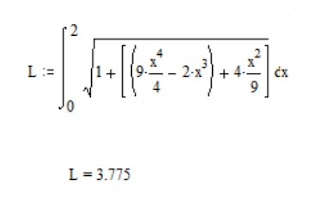## Wednesday, May 18, 2022

### Solutions To Differential Geometry Problems - Part 4

1)A curve is given in spherical coordinates i  by:

x 1 = t,    x 2 = arcsin 1/t,    x 3  =  (t 2 – 1) 1/2

Compute the length of the arc between t = 1 and t = 2

Soln.

(ds/ dt) 2 =    (dx1/ dt) 2  +  (x1 ) 2  (dx2/ dt) 2    +   (x1  sin x  22 (dx3/ dt) 2

And:

(dx1/ dt) 2  =   1

(dx1/ dt) 2    =   [ -1/  t2   Ö {1 – (  1/  t2  )} = 1/ t2  (t2  -   1)

(dx3/ dt) 2    = 2t/ Ö2(t2  -   1) =  t/ (t2  -   1)

Whence:

(ds/ dt)2 =

1 +  t2   · 1/ t2  (t2  -   1) + (t ·  1/  t ) 2   ·  t/ (t2  -   1)

=   2 t2 / (t2  -   1)

Then the length of the curve is:

L = ò 2   Ö2 t/ (t2  -   1)1/2  dt = Ö2(t2  -   1) ] 12      =  Ö6

2) Obtain the arc length s of the curve:

f(x) =  x3 / 2   -   x

Between x = 0 and x = 2

Soln.

f(x) =  x3 / 2   -   x2 / 3

d(f(x) / dx =   3 x2 / 2 -  2x/ 3

(d(f(x) / dx) 2 =  (3 x2 / 2 -  2x/ 3 ) (3 x2 / 2 -  2x/ 3 )

=    9 x4 / 4  -   2 x3    +   4 x2 / 9

Then:

L  =  ò 0   Ö(1 +  9 x4 / 4  -   2 x3    +   4 x2 / 9)

=  3.775 units3) Determine the arc length of a catenary with parametric representation:  x(t) =  (t,  a cosh (t/a), 0)

Soln.:

Using the arc length computation equation we have:

x'(t) =  1, sinh (t/a), 0

And

x' · x' = 1  +  sinh 2 (t/a) =  cosh 2 (t/a)

But:  Öcosh 2 (t/a)  =   cosh  (t/a)

So:  s (t)  =   ò 0  cosh  (u/a) du =  a sinh(t/a)

where s = 0 corresponds to t = 0

4) Find the full arc length of the Archimedian spiral shown by changing the integral to the correct limits.

Soln.

By inspection of the graph for r  =  q - sin  qwe need to have  the limits of integration from:    0  to  5.2 p/2:

Then: L  = 2.545  units, e.g. from Mathcad computation: